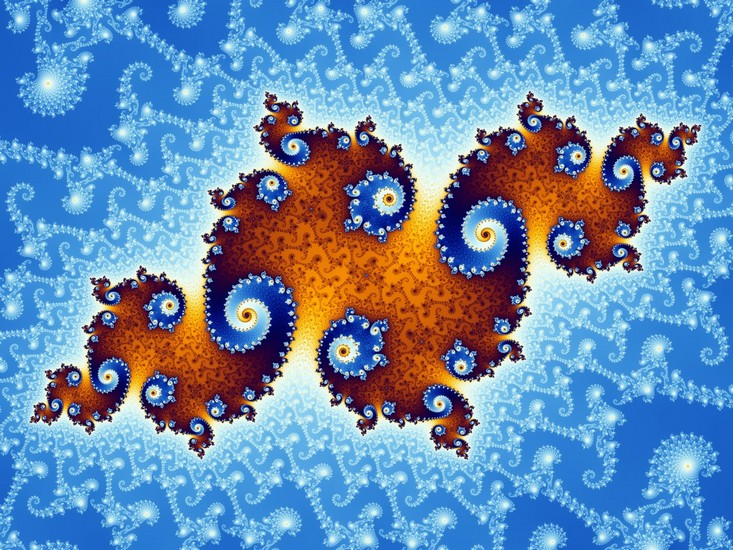Fractal art represents the alliance of art and mathematics. This recent art form uses computers to make images from mathematical formulas. Fractal art has an often geometric appearance, intricate patterns and a wealth of detail. But how do you go about creating images from mathematical formulas ?

## First of all, what does “Fractal” mean ?

In the 1970s, computer scientist Benoit Mandelbrot discovered one of the most famous fractal images: the Mandelbrot set.

This discovery is made possible by the development of computer, in particular thanks to the computing power. It also takes place in the scientific context of the development of the Chaos theory which studies dynamic phenomena (turbulence, vortices in a fluid, oscillation, cloud shape, etc.).

Likewise, fractal geometry is found in the shapes of nature with complex contours (snowflake, leaf of a tree, shape of clouds, eddies, arborescence of the blood network, shape of maritime coasts, etc.) that it was difficult to understand, and to model with the previous mathematical tools.

## Characteristics of fractal images

### The fractal dimension

The term “fractal” refers to the idea of ​​a non-whole, fractional dimension. Until the 1960s, mathematics studied decomposable objects using integer dimensions:

a point: dimension 0

a line: dimension 1

a plan: dimension 2

a volume: dimension 3

But imagine a sheet of paper. It represents a plane, therefore has a dimension equal to 2. If we crumple this sheet, it now occupies a volume (dimension 3), but is not a volume because we cannot “fill” the crumpled sheet with a material as one would fill a container. The dimension of the crumpled sheet is therefore between 2 and 3 : 2.568 for example. A fractal dimension ! Fractal objects thus have complex, irregular shapes, with contours folded and folded in on themselves.

### Self-similarity

Despite its complexity, a fractal object exhibits similar details. Zooming inside a fractal shows the same overall pattern repeated at different scales, and into infinity. A part is therefore similar to the whole. This property is called scale invariance.

## Generation of fractal images, “Simplicity breeds complexity”

The construction of fractal images is based on the use of Algorithm executed in a loop. It is an iterative process repeating a series of simple operations.

### Fractals by recurrence

For each point in space, the coordinates of the point are passed into an equation. The result is then fed back into the same equation. The operation is performed several times in a row (iterations). Then we test the result. If the result tends to infinity, then the starting point is not in the whole : it is colored white. If the result remains stable, or periodic, then the starting point is in the whole : it is colored black. For points on the boundary, it is more difficult to determine whether they are in the set or outside. It is then necessary to carry out more iterations. Depending on the number of iterations required to determine the point, it is colored in different shades. The points of this border give to the fractal its complicated and tangled appearance.

To draw the Mandelbrot set, we use complex numbers that each represent a pixel on the plane. We execute the following equation for each point : Zn + 1 = Zn2 + C, with Z0 = 0.

### IFS, iterated functions systems

Image construction is done here by copying itself. But with each copy, the copied image undergoes a geometric transformation (a linear transformation represented in mathematics by a linear function). These transformations can be rotations, flattening, shearing … The copies are also contracted before being placed back on the original image. The process is repeated until an image is formed.

This example results in the image of the Sierpinski triangle.

Another example is the generation of the Koch snowflake.

IFS can easily produce images reminiscent of natural objects, such as trees, ferns, etc.